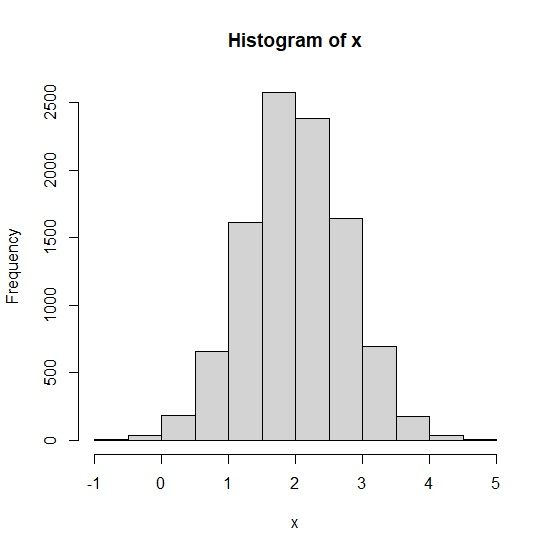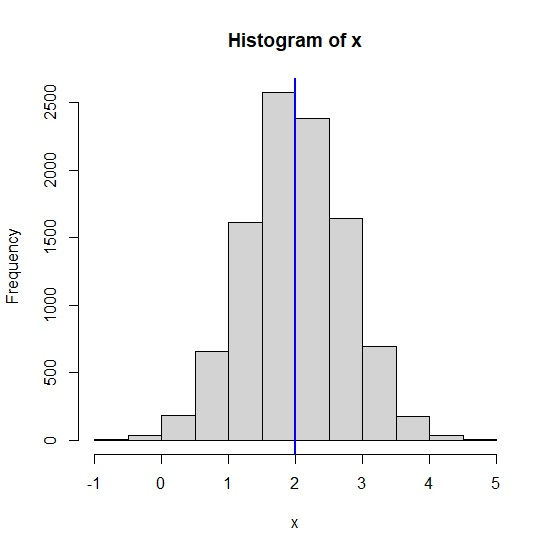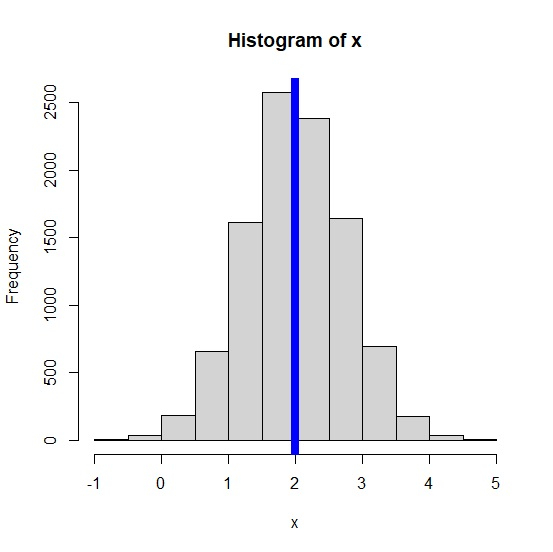# How to represent the mean with vertical line in a histogram created by hist function in R?

The value of mean is an important characteristic of the data to be represented by a histogram, therefore, one might want to plot it with the histogram. If the histogram is created by using hist function then we can create a vertical line on the histogram with the help of abline function by defining mean of the data for vertical argument v.

## Example

set.seed(101)
x<-rnorm(10000,2,0.75)
hist(x)

## OutputAdding a blue color line at the mean value of x in the histogram −

abline(v=mean(x),col="blue",lwd=2)

## Outputabline(v=mean(x),col="blue",lwd=8)

## Output